# What can we infer from proportional hazards?

Colleagues and I recently wrote a letter to the editor regarding difficulties of interpreting period specific hazard ratios from randomised trials as representing solely changes in treatment effect over time, as discussed in a previous post. The authors whose paper we were writing about responded to our letter. One of their points was the following:

Note that in a randomized controlled trial, if the proportional hazards assumption holds and there are no unobserved confounders (i.e., the patient population is homogeneous and there is no differential treatment effect across different subpopulations), a HR generated by Cox regression with treatment alone as a single covariate does have a causal interpretation. The hazard functions in this case do not depend on any confounder.

It is fairly implausible in any setting that a patient’s hazard does not depend on any of their characteristics. Indeed it is because we often have some understanding of what these variables are that they are used in stratified randomisation schemes and in the statistical analysis of the trial’s data.

In this post I will follow-up a little bit on this point, in terms of what can be concluded when the hazard ratio comparing treatment groups appears to be constant over time in a randomised trial. While if the estimated hazard ratio varies over the follow-up, there may be difficulties in causally interpreting the hazard ratio from different periods, surely if the hazard ratio seems to be constant, we should be able to legitimately interpret this as saying that for patients in the active arm, their hazard is multiplied by a time-constant factor (the HR we estimate) compared to if they were on the control treatment? Unfortunately this is not the case. We will perform a simulation where for each patient, the causal hazard ratio, i.e. the ratio of their individual hazard under active treatment compared to control, varies over time, despite the observable HR being constant over time. To do this, we will simulate under the frailty model described in the supplementary materials of the paper by Stensrud et al 2019.

To describe the setup, suppose we have randomly assigned patients to control or active treatment. We assume the existence of a frailty variable Z which encapsulates patient characteristics which influence time to the event of interest. For mathematical convenience in this illustration, we assume Z is gamma distributed across the population with mean 1 and variance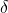. For patients on the control arm, we assume their hazard function, conditional on the value of their frailty variable Z, is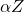for some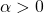. For patients in the active arm, we assume their hazard is equal to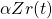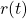thus controls the hazard ratio for active compared to control for those patients with frailty equal to Z. In a randomised trial we measure some baseline covariates, but we don’t measure all of the variables which contribute to between patient frailty. Thus in practice Z is not observed. The observable/estimable hazard ratio at a given time comparing active to control patients is depends on– see the supplementary materials of Stensrud et al 2019 for the details. But in order to construct this example to make our point, we choosesuch that the observable hazard ratio (not conditional on Z) is constant over time. Stensrud et al show that to do this we needto obey: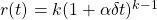Under this setup, the event times in the control arm are exponentially distributed conditional on the frailty variable Z. A bit more algebra can be derived to show what the survival function is for the active arm patients, conditional on Z, which will enable us to simulate data under these assumptions. The following R code simulates data for a very large trial under these assumptions, with particular values of the parameters. The marginal/observable HR we specify is 0.6, which is the HR we should expect to estimate from the analysis when Z is unmeasured.

library(survival)
n <- 100000
delta <- 2
alpha <- 1
k <- 0.6
set.seed(6234787)

#simulate controls
z_c <- rgamma(n,scale=delta,shape=1/delta)
t_c <- rexp(n,rate=alpha*z_c)
#simulate active treatment. Note frailty distribution is identical in both arms
z_a <- rgamma(n,scale=delta,shape=1/delta)
u <- runif(n)
t_a <- ((1-alpha*delta*log(u)/z_a)^(1/k)-1)/(alpha*delta)

simData <- data.frame(trt=c(rep(0,n), rep(1,n)), t=c(t_c, t_a))
#impose some uniform censoring
maxFup <- 5
simData$c <- maxFup*runif(n*2) simData$delta <- 1*(simData$t < simData$c)
simData$v <- simData$t
simData$v[simData$delta==0] <- simData$c[simData$delta==0]

Now that we have simulated our extremely large trial, let’s take a look at the Kaplan-Meier survival curves:

km <- survfit(Surv(v,delta)~trt, data=simData)
plot(km, col=c("red","blue"))
legend(4,0.8,legend=c("Control","Active"), fill = c("red","blue", lwd=c(2,2)))


Next we fit a Cox model to the data with the patient’s treatment group as covariate:

coxmod <- coxph(Surv(v,delta)~trt, data=simData)
summary(coxmod)
Call:
coxph(formula = Surv(v, delta) ~ trt, data = simData)

n= 200000, number of events= 91290

coef exp(coef)  se(coef)      z Pr(>|z|)
trt -0.520104  0.594458  0.006738 -77.19   <2e-16 ***
---
Signif. codes:  0 ‘***’ 0.001 ‘**’ 0.01 ‘*’ 0.05 ‘.’ 0.1 ‘ ’ 1

exp(coef) exp(-coef) lower .95 upper .95
trt    0.5945      1.682    0.5867    0.6024

Concordance= 0.564  (se = 0.001 )
Likelihood ratio test= 6078  on 1 df,   p=<2e-16
Wald test            = 5959  on 1 df,   p=<2e-16
Score (logrank) test = 6093  on 1 df,   p=<2e-16

We have an estimated HR (labelled exp(coef)) of 0.59, very close to the value of 0.6 used in the simulation process. They should be clos because we have simulated with such a large dataset. Next let’s plot how the log HR is estimated to vary over time, based on the Schoenfeld residuals:

plot(cox.zph(coxmod, transform="identity"))

This plot, as expected, shows that the hazard ratio (marginal to Z) is constant over time. So the proportional hazards assumption we are able to check from the observable data seems reasonable, which here we know is correct because of the way we’ve simulated data. But now let’s look at how the true individual level HR changes over time, based on the earlier equation for:

tval <- seq(0,maxFup,0.01)
rt <- k*(1+alpha*delta*tval)^(k-1)
plot(tval,rt)

This shows that conditional on Z, i.e. for a given individual, the HR changes quite dramatically over time (for as long as they are still at risk). In particular, here the effect is increasing over time, with the HR moving farther away from 1. To show this empirically, let’s re-run the simulation, but set everyone’s value of the frailty variable Z to the same value of 1. Thus the trial represents a situation where the patients are completely homogeneous in respect of their hazard functions. The code below is identical to before, except we’ve modified the line which previously simulated the frailty values from a gamma distribution, so that they now are equal to 1 for all patients.


#re-run with Z=1 for all
#simulate controls
z_c <- rep(1,n)
t_c <- rexp(n,rate=alpha*z_c)
#simulate active treatment. Note frailty distribution is identical in both arms
z_a <- rep(1,n)
u <- runif(n)
t_a <- ((1-alpha*delta*log(u)/z_a)^(1/k)-1)/(alpha*delta)

simData <- data.frame(trt=c(rep(0,n), rep(1,n)), t=c(t_c, t_a),z=c(z_c,z_a))
#impose some uniform censoring
maxFup <- 5
simData$c <- maxFup*runif(n*2) simData$delta <- 1*(simData$t < simData$c)
simData$v <- simData$t
simData$v[simData$delta==0] <- simData$c[simData$delta==0]

km <- survfit(Surv(v,delta)~trt, data=simData)
plot(km, col=c("red","blue"))
legend(4,0.8,legend=c("Control","Active"), fill = c("red","blue", lwd=c(2,2)))
coxmod <- coxph(Surv(v,delta)~trt, data=simData)
summary(coxmod)
plot(cox.zph(coxmod, transform="identity"))

Here I’ll just show the final plot, which is showing the estimated log HR over time among the new trial patients (for whom there is no heterogeneity in frailty):

As expected from the theory, among this now homogeneous group of patients, the HR is decreasing over time, corresponding to the effect (on the hazard ratio scale) increasing over time. The differences between the analysis conditional on Z and that averaged/marginal to Z are due to the fact that as time goes on, the survivors (risk sets) in the two treatment groups at some time t>0, who are used to estimate the HR at t, have different distributions of the frailty variable Z. As such, the observable/estimable HR at some time t>0 is confounded by Z.

This example is not intended to suggest the form for how the individual level HR changes over time is realistic for any given setting. Rather it is simply intended to demonstrate that one can have proportional hazards in terms of what can be observed in the trial even when at the individual level the hazard ratio is changing over time. In conclusion, as described by Stensrud et al 2019, the hazard ratios we can actually estimated from trials (or indeed observational data) are difficult to interpret causally, even when the proportional hazards assumption that we can actually check seems ok.

### 1 thought on “What can we infer from proportional hazards?”

1.Dominic Magirr asked on Twitter (https://twitter.com/DominicMagirr/status/1324641981994336261?s=20) about whether an individual hazard function really exists. This is an excellent question, to which I don’t know the answer! The question is about whether about all patient level characteristics, both measured and unmeasured, in all their complexity, determines the time to event, or whether there remains residual randomness. There is an excellent discussion of this point in the first few pages of Chapter 6 in the book ‘Survival and Event History Analysis’ by Aalen, Borgan and Gjessing. Their view (I believe) is that it is reasonable to conceive of an individual hazard function, corresponding to the idea that outcomes are stochastic rather than deterministic. I also discussed this a bit in a talk I gave at ISCB two years ago, the slides of which are here: http://thestatsgeek.com/wp-content/uploads/2018/08/ISCB_causalHR_2018_08_29.pdf

In practice I suspect that most people interpret HRs from Cox models as meaning that if a given patient takes the new treatment, their hazard will be multiplied by HR compared to what it would have been if they had taken the control. As such they are I think implicitly assuming the existence of an individual level hazard. Similarly, I suspect many people interpret an odds ratio from a trial as the effect on the patient level, even though it is a marginal effect. Indeed with a binary outcome, if one believes potential outcomes are deterministic, the risk difference for an individual can only be one of +1, 0, -1.

If one doesn’t like the idea of an individual hazard function, then I think the correct way to interpret the marginal HR (assuming it is constant over time) is as Dominic suggests on Twitter as a population level effect on the hazard. There is some further discussion on an alternative interpretation in this conference presentation and this previous blog post.